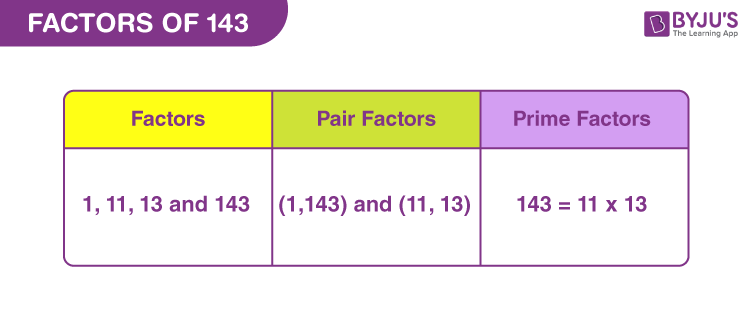# Factors of 143

In Mathematics, factors of 143 are the real numbers that evenly divide the original number. 143 is a composite number, since it has more than two factors. We can find these factors easily by dividing 143 by the natural numbers. For example, if 45 divided by 9 is 5, then 9 is the factor of 45.

Now, let us find the factors, prime factors and pair factors of 143 in this article.## How to Find Factors of 143?

Factors are the integers that divide the original number (in this case, 143), evenly or equally.

By dividing 143 by the sequence of natural numbers, we can find the required factors. If the quotient produced, after division, is a whole number, then the divisor is the factor.

143 ÷ 1 = 143

143 ÷ 11 = 13

143 ÷ 13 = 11

143 ÷ 143 = 1

Thus, the factors of 143 are 1, 11, 13 and 143.

## Pair Factors of 143

Pair factors are those numbers in pairs that result in the original number (in this case 143) when multiplied together.

1 × 143 = 143

11 × 13 = 143

Therefore, the pair factors are (1, 143) and (11, 13).

Now, if we consider the negative pair factors, then;

-1 × -143 = 143

-11 × -13 = 143

Therefore, the negative pair factors are (-1, -143) and (-11, -13).

## Prime Factorisation of 143

Prime factors of 143 divide the original number into equal parts. The list of prime numbers from 1 to 150 is given below. So, here we need to find such prime numbers that can divide 143 completely.

List of prime numbers (1 to 150) :

 2, 3, 5, 7, 11, 13, 17, 19, 23, 29, 31, 37, 41, 43, 47, 53, 59, 61, 67, 71, 73, 79, 83, 89, 97, 101, 103, 107, 109, 113, 127, 131, 137, 139, 149

Step 1: Since 143 is an odd number, so we cannot divide it by 2. Now 143 is directly divisible by 11.

143/11 = 13

Step 2: Now 13 is itself a prime number. Thus,

13/13 = 1

Therefore,

 Prime factorisation of 143 = 11 x 13

## Solved Examples

Q.1: If 11 tennis balls are bought for Rs.143. Then what is the cost of each tennis ball?

Solution: Given,

Number of balls bought = 11

Cost of 11 balls = Rs.143

Each ball will cost = 143/11 = 13

Therefore, cost of each ball is Rs.13.

Q.2: Find the sum of all the factors of one hundred and forty-three.

Solution: The factors of 143 are 1, 11 ,13 and 143.

Sum = 1 + 11 + 13 + 143 = 168

Therefore, 168 is the required sum.

Q.3: What is the greatest common factor of 145 and 143?

Answer: Let us write the factors of both the numbers.

143 → 1, 11, 13, 143

145 → 1, 5, 29, 145

Hence, the greatest common factor is only 1.

Q.4: What is the GCF of 143 and 156?

Solution: We know the factors of 143 are 1, 11, 13 and 143.

Factors of 156 = 1, 2, 3, 4, 6, 12, 13, 26, 39, 52, 78, 156

If we compare the factors, 13 is the greatest common factor of 143 and 156.

GCF (143, 156) = 13

## Frequently Asked Questions on Factors of 143

### 1. What are the factors of 143?

Answer: There are a total of four factors of 143. These factors are 1, 11,13 and 143.

### 2. What are the multiples of 143?

Answer: The first 10 multiples of 143 are 143, 286, 429, 572, 715, 858, 1001, 1144, 1287 and 1430.

### 3. How is 143 represented as the product of prime factors?

Answer: The prime factorisation of 143 is:

143 = 11 x 13

### 4. Is 5 a factor of 143?

Answer: No, 5 is not a factor of 143. 5 cannot divide 143 evenly.

### 5. Is 143 a composite number?

Answer: Yes, 143 is a composite number because it has more than 2 factors.

### 6. Is 143 a perfect square?

Answer: 143 is not a perfect square because the root of 143 will result in a fraction.

√143 = 11.96 (approximately)